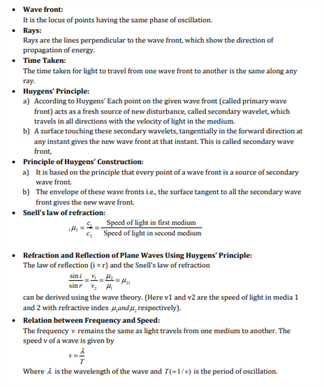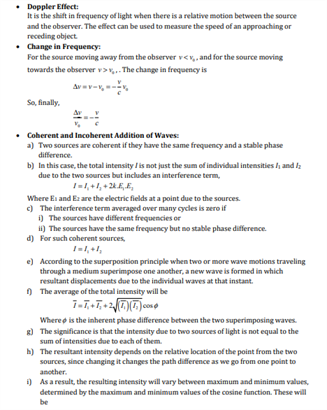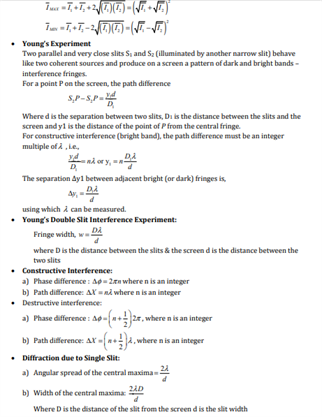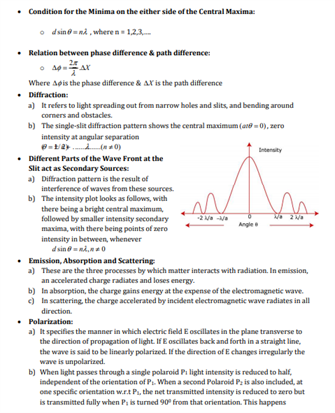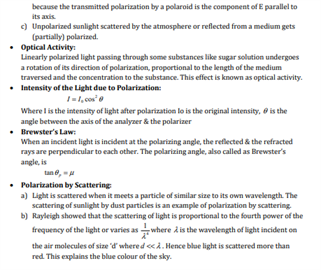# Physics Revision Notes for Class 12 for Chapter 10 Wave Optics

In 1678, Christiaan Huygens, a Dutch physicist, put forward the wave theory of light. This wave model satisfactorily explained the phenomena of reflection and refraction. It predicted that on refraction if the light wave bends towards the normal then the speed of light would be less in the second medium. The wave theory of light is mainly discussed in this chapter. The following are the topics that we will be studying in this chapter:

• The original formulation of Huygen’s principle and the derivation of laws of reflection and refraction
• The phenomenon of interference based on the principle of superposition
• The phenomenon of diffraction based on the HuygensFresnel principle
• The phenomenon of polarisation based on the fact that the light waves are transverse electromagnetic waves

Available here is a concise CBSE class 12 physics revision notes for chapter 10 wave optics.

Available below is the revision notes for chapter 10 of class 12 physics.